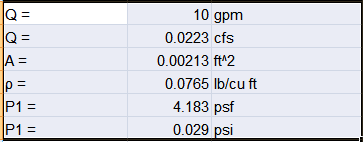# Air Displacement Pressure

I am struggling with what seemed to be a simple problem and any help would be greatly appreciated.
I have to determine the amount of pressure created when air is displaced buy gasoline flowing into a tank.
The gas enters the tank at 10gpm. The entrance has a diameter of 1.5" and the vent has a diameter of 5/8".
I need to know the pressure of the air at the exit of the tank.
Can this be modeled with the Bernoulli equation?
Using the Bernoulli equation I got an answer of around 2psi but this does not seem quite right.

russ_watters
Mentor
Yes, I would think Bernoulli's would work.

2psi seems high. Can you show your work?

Here's what I've got.

#### Attachments

•20180817_080727_HDR.jpg
25.8 KB · Views: 252
JBA
Gold Member
Caleb, you want the entrance pressure to the vent, which is also the tank static pressure, with an vent air volume flow equivalent to that of the gasoline volume flow into the tank.

The simplest Bernoulli's equation for that determination reduces to: P1 = .5*ρ*(Q/A)^2, With ρ in lb/cu ft, Q in cfs, and A in ft^2 for units consistency.

In that equation, P1 is the static pressure in the tank, and .5*ρ*Q/A is the equivalent amount of dynamic flowing energy thru the vent that can be converted from the P1 tank static pressure.
The result will be a tank pressure in psf that when converted to psi is much less than 1 psi.

Last edited:
I also attempted to use the fluid momentum equation which gave me approximately .06 psig but I was very unsure about the validity of this principle in this particular situation. I was also able to use water that had a flow rate of 7 gpm and determine the pressure experimentally and it was about .75 psig. I assume this is very similar to the number I would get with the gas except the gas would create less pressure because it has a lower density.

JBA
Gold Member
Using my above equation and units, my result for air is:#### Attachments

•russ_watters